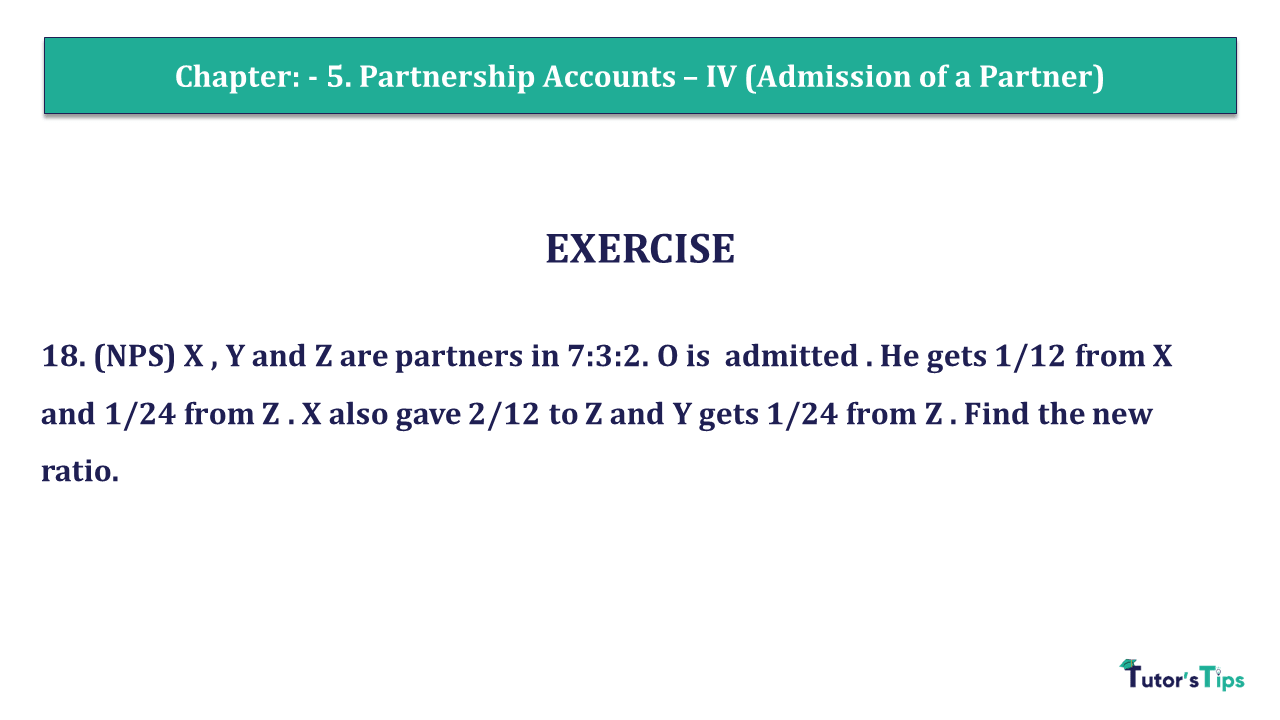# Question 18 Chapter 5 of +2 Part-1 – USHA Publication 12 Class Part – 1Q-18- CH-5 - Usha +2 Book 2018 - Solution

Question 18 Chapter 5 of +2-Part-1

18. (NPS) X , Y and Z are partners in 7:3:2. O is admitted . He gets 1/12 from X and 1/24 from Z . X also gave 2/12 to Z and Y gets 1/24 from Z . Find the new ratio.

We are providing a solution of Question 18 Chapter 5 of +2 Part-1 in two formats. one is in Video format and another is in article format. Check out both formats as follows:

### 1. Check out the Solution of this question in Video Format:-

The video consists solution of question numbers from 12 to 23 Chapter no. 5 class 12 of Usha publication. To check the direct solution of question no. 18 from the flowing video by using time stamps of the video.

## The solution of Question 18 Chapter 5 of +2 Part-1: –

Calculation of new Profit Sharing ratio

Old Profit Sharing ratio of X , Y and Z = 7:3:2

 X’s Sacrifice = 1 to O and 2 to Z 12 12
 X’s new share = 7 – 1 + 2 12 12 12 = 4 or 8 12 24 = 1 Z’s sacrifice for Y 24

Z’s Share = Old Share + Acquired from Z – ( Sacrifice for O Sacrifice for Y )

 Z’s new share = 2 – 2 – 1 : 1 12 12 24

 X’s sacrifice for B = 8 – 2 24 = 6 24
 Y’s new share = 3 + 1 12 24 = 7 24
 O’s share = 1 + 1 12 24 = 3 24
 New Profit sharing ratio = 8 : 7 : 6 : 3 24 24 24 24 New Profit sharing Ratio = 8 : 7 : 6 : 3

Comment if you have any questions.

Also, Check out the solved question of previous Chapters: –